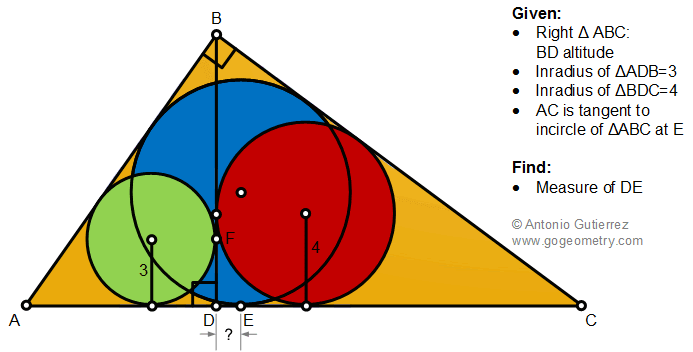## Friday, November 27, 2020

### Geometry Problem 1486: Right Triangle, Altitude, Incircle, Tangent, Measurement

Geometry Problem 1486. Post your solution in the comment box below.
Level: Mathematics Education, K-12 School, Honors Geometry, College.

Details: Click on the figure below.1.Tr.s ABD, BDC, ABC are similar.
If r is the inradius, r/b = 3/c = 4/a ( = 1/n say) and since a^2 + c^2 = b^2, r = 5.

So a = 4n, b = 5n and c = 3n

Now also r = ac/(a+b+c) from which 5.(4n + 5n + 3n) =12.n^2
so that n = 5 and hence a = 20, b = 25 and c = 15

So DE = CD - CE = 20^2/25 - (20 + 25 - 15)/2 = 16 - 15 = 1

Sumith Peiris
Moratuwa
Sri Lanka

2.From D are drown vertical two equal tangential segments
So FP (P tg point of red circle)=DE=4-3=1

1.How is FP = DE?

2.https://photos.app.goo.gl/9nhsrsHvmvmBh5HAA

3.So centers of three circles lie on a circle with center E
=> from one of righttriangles with side 3, 4 can calculate the R=5

3.Credits to Sumith sir for deriving InRadius = 5 and AB=15,BC=20,AC=25
BD.25=15.20
BD=12
let P,O,Q be the centers of Green,Blue and Red incircles.M,N be the foot of perpendiculars from P and Q on AC
let AM=u, CN=v and we know DM=3, DN=4
APM similar to AOE, so AE=5u/3
CQN similar to COE, so CE=5v/4
AE+CE=(20u+15v)/12=25
4u+3v=60
But u+3+v+4=25=> u+v=18
hence u=6 & AE=10

Another solution (using 1487):
Let X be on AD such that BX is the angle bisector of ABD
DX/XA=12/15=4/5
XA=5DX/4
So DX=4
EX=Inradius=5 (O center of Blue circle. BOXA is concyclic and triangle OXE is right and isosceles)
=>DE=EX-DX=1

4.ABD and BCD are similar and their sides are in the ratio 3:4
Apply Pythagoras to ABC
=>9/16x2+x2=25.25/16.16y2
=>x=5/4y
Substituting x with the above value and representing the sides of ABC in terms of y => a=5/4y, b=25/16y,c=15/16y
Semi-perimeter of ABC P=15/8y
AE=P-a=15/8y-5/4y=5/8y